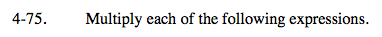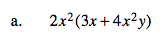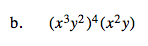### Home > A2C > Chapter 4 > Lesson 4.2.1 > Problem4-75

4-75.
1. Multiply each of the following expressions. Homework Help ✎

1. 2x2(3x + 4x2y)

2. (x3y2)4(x2y)6x3 + 8x4yRemember that the 4th power means to use the expression as a multiplier four times.

(x12y8)(x2y)

How can you rewrite this using the Commutative and Associative Properties of Multiplication to simplify it further?